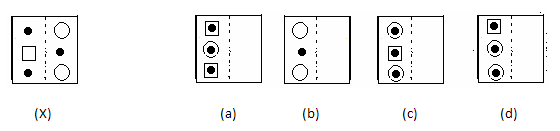# Paper Folding - Non Verbal Reasoning

## Paper Folding - Non Verbal Reasoning

1. Find out from amongst the four alternatives (a), (b), (c), (d), as to how the pattern would appear when the transparent sheet(X) is folded at the dotted line.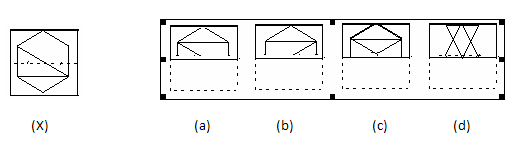2. Find out from amongst the four alternatives (a), (b), (c), (d), as to how the pattern would appear when the transparent sheet(X) is folded at the dotted line.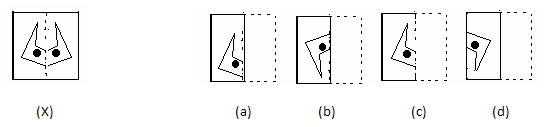3. Find out from amongst the four alternatives (a), (b), (c), (d), as to how the pattern would appear when the transparent sheet(X) is folded at the dotted line.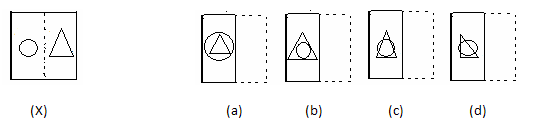4. Find out from amongst the four alternatives (a), (b), (c), (d), as to how the pattern would appear when the transparent sheet(X) is folded at the dotted line.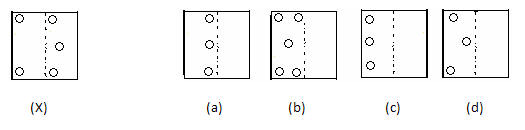5. Find out from amongst the four alternatives (a), (b), (c), (d), as to how the pattern would appear when the transparent sheet(X) is folded at the dotted line.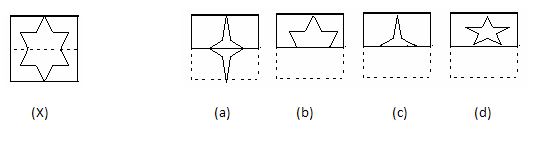6. Find out from amongst the four alternatives (a), (b), (c), (d), as to how the pattern would appear when the transparent sheet(X) is folded at the dotted line.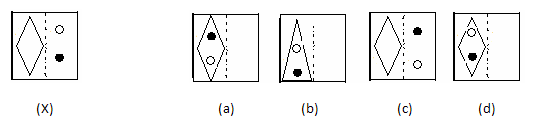7. Find out from amongst the four alternatives (a), (b), (c), (d), as to how the pattern would appear when the transparent sheet(X) is folded at the dotted line.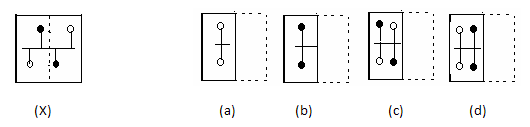8. Find out from amongst the four alternatives (a), (b), (c), (d), as to how the pattern would appear when the transparent sheet(X) is folded at the dotted line..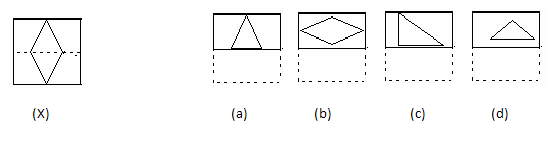9. Find out from amongst the four alternatives (a), (b), (c), (d), as to how the pattern would appear when the transparent sheet(X) is folded at the dotted line.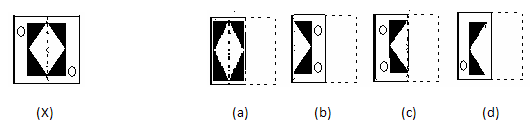10. Find out from amongst the four alternatives (a), (b), (c), (d), as to how the pattern would appear when the transparent sheet(X) is folded at the dotted line.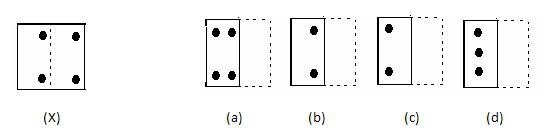11 Find out from amongst the four alternatives (a), (b), (c), (d), as to how the pattern would appear when the transparent sheet(X) is folded at the dotted line.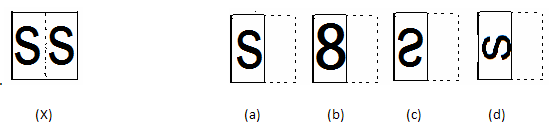.

12. Find out from amongst the four alternatives (a), (b), (c), (d), as to how the pattern would appear when the transparent sheet(X) is folded at the dotted line.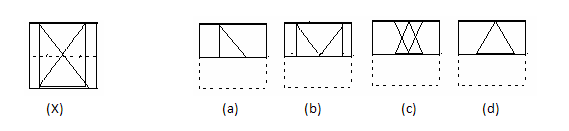13. Find out from amongst the four alternatives (a), (b), (c), (d), as to how the pattern would appear when the transparent sheet(X) is folded at the dotted line.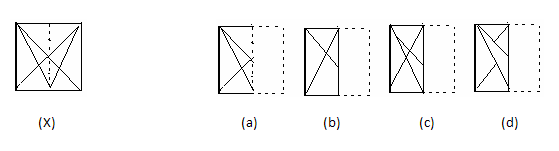14. Find out from amongst the four alternatives (a), (b), (c), (d), as to how the pattern would appear when the transparent sheet(X) is folded at the dotted line.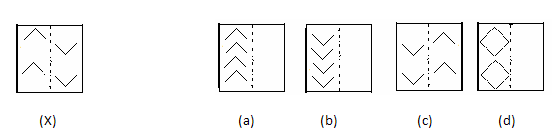15. Find out from amongst the four alternatives (a), (b), (c), (d), as to how the pattern would appear when the transparent sheet(X) is folded at the dotted line.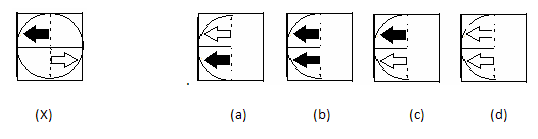.

16. Find out from amongst the four alternatives (a), (b), (c), (d), as to how the pattern would appear when the transparent sheet(X) is folded at the dotted line.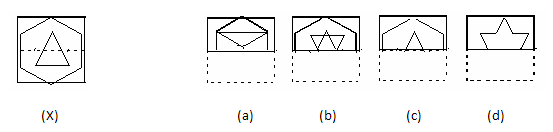17 Find out from amongst the four alternatives (a), (b), (c), (d), as to how the pattern would appear when the transparent sheet(X) is folded at the dotted line.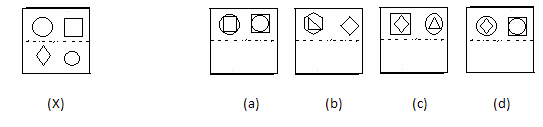18. Find out from amongst the four alternatives (a), (b), (c), (d), as to how the pattern would appear when the transparent sheet(X) is folded at the dotted line.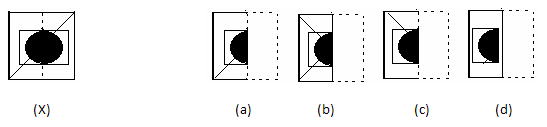19. Find out from amongst the four alternatives (a), (b), (c), (d), as to how the pattern would appear when the transparent sheet(X) is folded at the dotted line.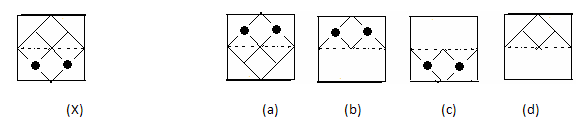20. Find out from amongst the four alternatives (a), (b), (c), (d), as to how the pattern would appear when the transparent sheet(X) is folded at the dotted line.